# Circle facts for kids

Kids Encyclopedia Facts
(Redirected from Circular)

A circle is a round, regular, two-dimensional shape, shaped like a 2D sphere

The centre of a circle is the point in the very middle.

The radius of a circle is a line from the centre of the circle to a point on the side.

All points on the edge of the circle are at the same distance from the centre. In other words, the radius is the same length all the way around the circle. Mathematicians use the letter r for the length of a circle's radius.

The diameter (meaning "all the way across") of a circle is a straight line that goes from one side to the opposite and right through the centre of the circle. Mathematicians use the letter d for the length of this line.

The diameter of a circle is equal to twice its radius (d equals 2 times r).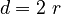$d = 2\ r$

The circumference (meaning "all the way around") of a circle is the line that goes around the centre of the circle. Mathematicians use the letter C for the length of this line.

The number π (written as the Greek letter pi) is a very useful number. It is the length of the circumference divided by the length of the diameter (π equals C divided by d). As a fraction the number π is equal to about 227 or 335/113 (which is closer) and as a number it is about 3.1415926535.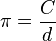$\pi = \frac{C}{d}$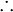$\therefore$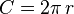$C = 2\pi \, r$

The area, a, inside a circle is equal to the radius multiplied by itself, then multiplied by π (a equals π times r times r).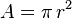$A = \pi \, r^2$

## Calculating π

π can be measured by drawing a large circle, then measuring its diameter (d) and circumference (C). This is because the circumference of a circle is always π times its diameter.$\pi = \frac{C}{d}$

π can also be calculated by only using mathematical methods. Most methods used for calculating the value of π have desirable mathematical properties. However, they are hard to understand without knowing trigonometry and calculus. However, some methods are quite simple, such as this form of the Gregory-Leibniz series: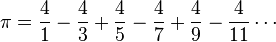$\pi = \frac{4}{1}-\frac{4}{3}+\frac{4}{5}-\frac{4}{7}+\frac{4}{9}-\frac{4}{11}\cdots$

While that series is easy to write and calculate, it is not easy to see why it equals π. An easier to understand approach is to draw an imaginary circle of radius r centered at the origin. Then any point (x,y) whose distance d from the origin is less than r, calculated by the pythagorean theorem, will be inside the circle: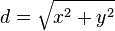$d = \sqrt{x^2 + y^2}$

Finding a set of points inside the circle allows the circle's area A to be estimated. For example, by using integer coordinates for a big r. Since the area A of a circle is π times the radius squared, π can be approximated by using: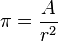$\pi = \frac{A}{r^2}$

## Images for kidsCircle Facts for Kids. Kiddle Encyclopedia.